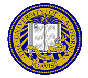# Sample Final

These are sample questions that are very similar to the ones I will ask on the midterm.

1. True or False: the value of isdigit(9) is 1.
2. True or False: In general, recursion is faster than iteration.
3. Multiple choice: I want to copy a string stored in an array src to another array called dest. Which of the following statements will do this?
1. dest = src;
2. dest[] = src[];
3. strcpy(dest, src);
4. while(*dest = *src);
5. strcmp(dest, src);
4. Multiple choice: Which of the following describes a recursive function?
1. It is a function with no parameters.
2. It is a function that contains loops to count something.
3. It is a function that calls itself.
4. It is a function that returns a value.
5. It is a function that is called to end the program.
5. Here is a macro for computing the maximum of two numbers:
```#define max(x, y)	((x) > (y) ? (x) : (y))
```
and here is a function:
```
int max(int a, int b)
{
return(a > b ? a : b);
}
```
In the following code fragment, give the values of a, b, c, d, and e at the end if max is the above macro, and then if max is a function call:
```a = -1;
b = 0;
c = 1;
d = max(++a, b);
e = max(c++, b);
```
variablevalue if max is macrovalue if max is a function
a
b
c
d
e
6. Here is a very simple program which is designed to sum its arguments:
``````
#include
``````
int main(int argc, char *argv[])
{
int sum = 0;
int i;

for(i = 1; i < argc; i++)
sum += argv[i];
printf("%d\n", sum);

return(0);
}
``````
1. Suppose this program is invoked as
```
sum 1 2 3
```
What are the values of argc and the elements of argv? Draw a picture if that would help you indicate what the values are. Please note I want the value of argc, argv, and each element of argv, and so forth, either as a number or character, or as arrows in a picture (for addresses).
2. When I ran this programs, I got 2093422869. Why does the program print the incorrect value, and how would you fix it? Feel free to us any library functions you might find helpful. (If you cannot write the code, for partial credit simply say what you would do.)
7. Here is a function:
``````
int mystery(char a[])
{
register int i;

for(i = 0; a[i]; i++)
if (!isdigit(a[i]))
return(0);
return(1);
}
``````
1. What does the above function do? Its purpose can be stated in a succinct sentence; for partial credit, you can describe what each line does, but for full credit, you must state its function in one very short sentence.
2. Rewrite the function using pointers rather than an array.
8. Rewrite the following into a Linux command or commands that do not use temporary files.
``````
tr A-Z a-z < /usr/share/dict/words > /tmp/X
grep '^bananna\$' /tmp/X > /tmp/Y
wc -l /tmp/Y
``````
9. What is the value of n after the following code executes?
``````
char charray[] = "A-DF123";
int n, s = 1;
char *p;
``````
for(n = 0, p = charray; *p; p++){
switch(*p){
case '-':
s = -s;
break;
case '0':
case '1':
case '2':
case '3':
case '4':
case '5':
case '6':
case '7':
case '8':
case '9':
n = n * 10 + (*p - '0');
break;
}
}
n = s * n;
``````Matt Bishop Office: 2209 Watershed Sciences Phone: +1 (530) 752-8060 Email: mabishop@ucdavis.edu
ECS 36A, Programming and Problem Solving
Version of December 1, 2019 at 11:09PM